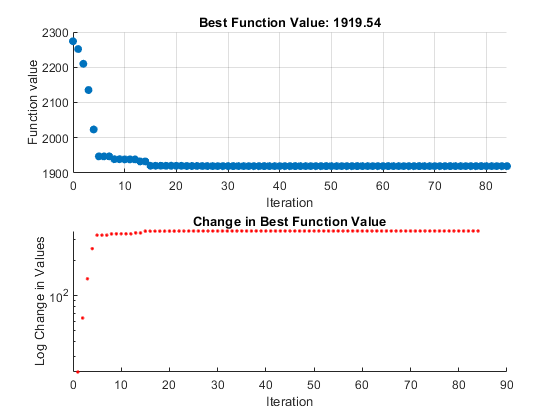Custom Plot Function

To use a plot function other than those included with the software, you can write your own custom plot function that is called at each iteration of the pattern search to create the plot. This example shows how to create a plot function that displays the logarithmic change in the best objective function value from the previous iteration to the current iteration. More plot function details are available in Plot Options.

Creating the Custom Plot Function

To create the plot function for this example, copy and paste the following code into a new function file in the MATLAB® Editor:

function stop = psplotchange(optimvalues, flag)
% PSPLOTCHANGE Plots the change in the best objective function
% value from the previous iteration.

% Best objective function value in the previous iteration
persistent last_best

stop = false;
if(strcmp(flag,'init'))
set(gca,'Yscale','log'); %Set up the plot
hold on;
xlabel('Iteration');
ylabel('Log Change in Values');
title(['Change in Best Function Value']);
end

% Best objective function value in the current iteration
best = min(optimvalues.fval);

% Set last_best to best
if optimvalues.iteration == 0
last_best = best;

else
%Change in objective function value
change = last_best - best;
plot(optimvalues.iteration, change, '.r');
end

Save the file as psplotchange.m in a folder on the MATLAB path. The code is explained in How the Plot Function Works.

Set Up the Problem

The problem is the same as Constrained Minimization Using patternsearch and Optimize Live Editor Task. To set up the problem:

1. Enter the following at the MATLAB command line:

x0 = [2 1 0 9 1 0];
Aineq = [-8 7 3 -4 9 0];
bineq = 7;
Aeq = [7 1 8 3 3 3; 5 0 -5 1 -5 8; -2 -6 7 1 1 9; 1 -1 2 -2 3 -3];
beq = [84 62 65 1];
2. Because this is a linearly constrained problem, set the PollMethod option to 'GSSPositiveBasis2N'. Include both the @psplotbestf built-in plot function and the custom plot function @psplotchange in the options.

options = optimoptions('patternsearch',...
'PlotFcn',{@psplotbestf,@psplotchange},...
'PollMethod','GSSPositiveBasis2N');

Run the Optimization with Custom Plot Function

Run the example by calling patternsearch starting from x0.

[x,fval] = patternsearch(@lincontest7,x0,...
Aineq,bineq,Aeq,beq,[],[],[],options);Because the scale of the y-axis in the lower custom plot is logarithmic, the plot shows only changes that are greater than 0.

How the Plot Function Works

The plot function uses information contained in the following structures.

• optimvalues — Current state of the solver, a structure

• flag — Current status of the algorithm, a character vector

The most important statements of the custom plot function, psplotchange.m, are summarized in the following table.

Custom Plot Function Statements

StatementDescription
persistent last_best

Creates the persistent variable last_best, the best objective function value in the previous generation. Persistent variables are preserved over multiple calls to the plot function.

set(gca,'Yscale','log')

Sets up the plot before the algorithm starts.

best = min(optimvalues.fval)

Sets best equal to the minimum objective function value. The field optimvalues.fval contains the objective function value in the current iteration. The variable best is the minimum objective function value. For a complete description of the fields of the structure optimvalues, see Structure of the Plot Functions.

change = last_best - best

Sets the variable change to the best objective function value at the previous iteration minus the best objective function value in the current iteration.

plot(optimvalues.iteration, change, '.r')

Plots the variable change at the current objective function value, for the current iteration contained inoptimvalues.iteration.

Global Optimization Toolbox DocumentationGet trial now